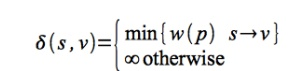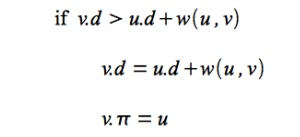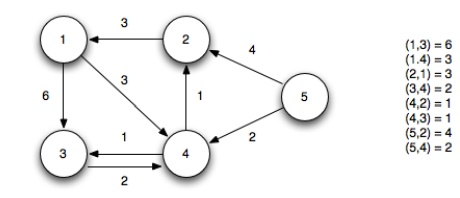Home | | Programming and Data Structure II | Bellman-Ford Algorithm

# Bellman-Ford Algorithm

Single Source Shortest Path

BELLMAN-FORD ALGORITHM:

Single Source Shortest Path

Problem

Given a directed graph G(V,E) with weighted edges w(u,v), define the path weight of a path p asFor a given source vertex s, find the minimum weight paths to every vertex reachable from s denotedThe final solution will satisfy certain caveats:

┬Ę        The graph cannot contain any negative weight cycles (otherwise there would be no minimum path since we could simply continue to follow the negative weight cycle producing a path weight of -Ōł×).

┬Ę        The solution cannot have any positive weight cycles (since the cycle could simply be removed giving a lower weight path).

┬Ę        The solution can be assumed to have no zero weight cycles (since they would not affect the minimum value).

Therefore given these caveats, we know the shortest paths must be acyclic (with Ōēż |V| distinct vertices) ŌćÆ Ōēż |V| - 1 edges in each path.

Generic Algorithm

The single source shortest path algorithms use the same notation as BFS with predecessor ŽĆ and distance d fields for each vertex. The optimal solution will have v.d =

╬┤(s,v) for all v Ōłł V.

The solutions utilize the concept of edge relaxation which is a test to determine whether going through edge (u,v) reduces the distance to v and if so update v.ŽĆ and v.d. This is accomplished using the conditionBellman-Ford Algorithm

The Bellman-Ford algorithm uses relaxation to find single source shortest paths on directed graphs that may contain negative weight edges. The algorithm will also detect if there are any negative weight cycles (such that there is no solution).

BELLMAN-FORD(G,w,s)

1.   INITIALIZE-SINGLE-SOURCE(G,s)

2.   for i = 1 to |G.V|-1

3.   for each edge (u,v) Ōłł G.E

4.      RELAX(u,v,w)

5. for each edge (u,v) Ōłł G.E

6.         if v.d > u.d + w(u,v)

7.               return FALSE

8. return TRUE

INITIALIZE-SINGLE-SOURCE(G,s)

1. for each vertex v Ōłł G.V

2.         v.d = Ōł×

3.         v.pi = NIL

4. s.d = 0

RELAX(u,v,w)

1.   if v.d > u.d + w(u,v)

2.         v.d = u.d + w(u,v)

3.         v.pi = u

Basically the algorithm works as follows:

1.     Initialize d's, ŽĆ's, and set s.d = 0 ŌćÆ O(V)

2.     Loop |V|-1 times through all edges checking the relaxation condition to compute minimum distances ŌćÆ (|V|-1) O(E) = O(VE)

3.     Loop through all edges checking for negative weight cycles which occurs if any of the relaxation conditions fail ŌćÆ O(E)

The run time of the Bellman-Ford algorithm is O(V + VE + E) = O(VE).

Note that if the graph is a DAG (and thus is known to not have any cycles), we can make Bellman-Ford more efficient by first topologically sorting G (O(V+E)), performing the same initialization (O(V)), and then simply looping through each vertex u in topological order relaxing only the edges in Adj[u] (O(E)). This method only takes O(V + E) time. This procedure (with a few slight modifications) is useful for finding critical paths for PERT charts.

Example:

Given the following directed graphUsing vertex 5 as the source (setting its distance to 0), we initialize all the other distances to Ōł×.Iteration 1: Edges (u5,u2) and (u5,u4) relax updating the distances to 2 and 4Iteration 2: Edges (u2,u1), (u4,u2) and (u4,u3) relax updating the distances to 1, 2, and 4 respectively. Note edge (u4,u2) finds a shorter path to vertex 2 by going through vertex 4Iteration 3: Edge (u2,u1) relaxes (since a shorter path to vertex 2 was found in the previous iteration) updating the distance to 1Iteration 4: No edges relaxThe final shortest paths from vertex 5 with corresponding distances isNegative cycle checks: We now check the relaxation condition one additional time for each edge. If any of the checks pass then there exists a negative weight cycle in the graph.

v3.d > u1.d + w(1,3) ŌćÆ 4 Ōē» 6 + 6 = 12 Ō£ō

v4.d > u1.d + w(1,4) ŌćÆ 2 Ōē» 6 + 3 = 9 Ō£ō

v1.d > u2.d + w(2,1) ŌćÆ 6 Ōē» 3 + 3 = 6 Ō£ō

v4.d > u3.d + w(3,4) ŌćÆ 2 Ōē» 3 + 2 = 5 Ō£ō

v2.d > u4.d + w(4,2) ŌćÆ 3 Ōē» 2 + 1 = 3 Ō£ō

v3.d > u4.d + w(4,3) ŌćÆ 3 Ōē» 2 + 1 = 3 Ō£ō

v2.d > u5.d + w(5,2) ŌćÆ 3 Ōē» 0 + 4 = 4 Ō£ō

v4.d > u5.d + w(5,4) ŌćÆ 2 Ōē» 0 + 2 = 2 Ō£ō

Note that for the edges on the shortest paths the relaxation criteria gives equalities. Additionally, the path to any reachable vertex can be found by starting at the vertex and following the ŽĆ's back to the source. For example, starting at vertex 1, u1.ŽĆ = 2, u2.ŽĆ = 4, u4.ŽĆ

= 5 ŌćÆ the shortest path to vertex 1 is {5,4,2,1}.

Study Material, Lecturing Notes, Assignment, Reference, Wiki description explanation, brief detail
Programming and Data structures : Graphs : Bellman-Ford Algorithm |